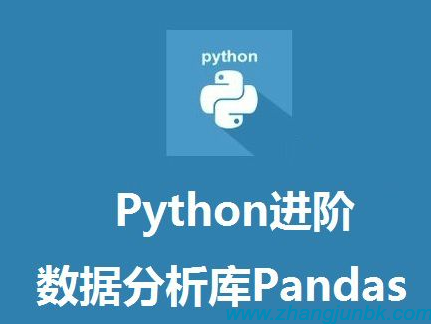# pandas常用函数### import语句

import pandas as pd

import numpy as np

import matplotlib.pyplot as plt

import datetime

import re

### 文件读取

names=['A', 'B', 'C'...] 自定义列名

index_col='A'|['A', 'B'...] 给索引列指定名称，如果是多重索引，可以传list

skiprows=[0,1,2] 需要跳过的行号，从文件头0开始，skip_footer从文件尾开始

nrows=N 需要读取的行数，前N行

sep=':'数据分隔默认是','，根据文件选择合适的分隔符，如果不指定参数，会自动解析

skip_blank_lines=False 默认为True，跳过空行，如果选择不跳过，会填充NaN

converters={'col1', func} 对选定列使用函数func转换，通常表示编号的列会使用（避免转换成int）

### 数据预处理

df.duplicated() 返回各行是否是上一行的重复行

df.drop_duplicates() 删除重复行，如果需要按照列过滤，参数选填['col1', 'col2',...]

df.fillna(0) 用实数0填充na

df.dropna() axis=0|1 0-index 1-column

how='all'|'any' all-全部是NA才删 any-只要有NA就全删

del df['col1'] 直接删除某一列

df.drop(['col1',...], aixs=1) 删除指定列，也可以删除行

df.column = col_lst 重新制定列名

df.rename(index={'row1':'A'}, 重命名索引名和列名

columns={'col1':'A1'})

df.replace(dict) 替换df值，前后值可以用字典表，{1:‘A', '2':'B'}

def get_digits(str):

m = re.match(r'(\d+(\.\d+)?)', str.decode('utf-8'))

if m is not None:

return float(m.groups())

else:

return 0

df.apply(get_digits) DataFrame.apply，只获取小数部分，可以选定某一列或行

df['col1'].map(func) Series.map，只对列进行函数转换

pd.merge(df1, df2, on='col1',

how='inner'，sort=True) 合并两个DataFrame，按照共有的某列做内连接（交集），outter为外连接（并集），结果排序

pd.merge(df1, df2, left_on='col1',

right_on='col2') df1 df2没有公共列名，所以合并需指定两边的参考列

pd.concat([sr1, sr2, sr3,...], axis=0) 多个Series堆叠成多行，结果仍然是一个Series

pd.concat([sr1, sr2, sr3,...], axis=1) 多个Series组合成多行多列，结果是一个DataFrame，索引取并集，没有交集的位置填入缺省值NaN

df1.combine_first(df2) 用df2的数据补充df1的缺省值NaN，如果df2有更多行，也一并补上

df.stack() 列旋转成行，也就是列名变为索引名，原索引变成多层索引，结果是具有多层索引的Series，实际上是把数据集拉长

df.unstack() 将含有多层索引的Series转换为DataFrame，实际上是把数据集压扁，如果某一列具有较少类别，那么把这些类别拉出来作为列

df.pivot() 实际上是unstack的应用，把数据集压扁

pd.get_dummies(df['col1'], prefix='key') 某列含有有限个值，且这些值一般是字符串，例如国家，借鉴位图的思想，可以把k个国家这一列量化成k列，每列用0、1表示

### 数据筛选

df.columns 列名，返回Index类型的列的集合

df.index 索引名，返回Index类型的索引的集合

df.shape 返回tuple，行x列

df.tail(n=M) 返回后M条

df.values 值的二维数组，以numpy.ndarray对象返回

df.index DataFrame的索引，索引不可以直接赋值修改

df.reindex(index=['row1', 'row2',...]

columns=['col1', 'col2',...]) 根据新索引重新排序

df[m:n] 切片，选取m~n-1行

df[df['col1'] > 1] 选取满足条件的行

df.query('col1 > 1') 选取满足条件的行

df.query('col1==[v1,v2,...]')

df.ix[:,'col1'] 选取某一列

df.ix['row1', 'col2'] 选取某一元素

df.ix[:,:'col2'] 切片选取某一列之前（包括col2）的所有列

df.loc[m:n] 获取从m~n行（推荐）

df.iloc[m:n] 获取从m~n-1行

df.loc[m:n-1,'col1':'coln'] 获取从m~n行的col1~coln列

sr=df['col'] 取某一列，返回Series

sr.values Series的值，以numpy.ndarray对象返回

sr.index Series的索引，以index对象返回

### 数据运算与排序

df.T DataFrame转置

df1 + df2 按照索引和列相加，得到并集，NaN填充

df - sr DataFrame的所有行同时减去Series

df * N 所有元素乘以N

sr.order() Series升序排列

df.sort_index(aixs=0, ascending=True) 按行索引升序

df.sort_index(by=['col1', 'col2'...]) 按指定列优先排序

df.rank() 计算排名rank值

### 数学统计

sr.unique Series去重

sr.value_counts() Series统计频率，并从大到小排序，DataFrame没有这个方法

sr.describe() 返回基本统计量和分位数

df.describe() 按各列返回基本统计量和分位数

df.count() 求非NA值得数量

df.max() 求最大值

df.min() 求最大值

df.sum(axis=0) 按各列求和

df.mean() 按各列求平均值

df.median() 求中位数

df.var() 求方差

df.std() 求标准差

df.cumsum() 求累计和

sr1.corr(sr2) 求相关系数

df.cov() 求协方差矩阵

df1.corrwith(df2) 求相关系数

pd.cut(array1, bins) 求一维数据的区间分布

pd.qcut(array1, 4) 按指定分位数进行区间划分，4可以替换成自定义的分位数列表

df['col1'].groupby(df['col2']) 列1按照列2分组，即列2作为key

df.groupby('col1') DataFrame按照列1分组

grouped.aggreagte(func) 分组后根据传入函数来聚合

grouped.aggregate([f1, f2,...]) 根据多个函数聚合，表现成多列，函数名为列名

grouped.aggregate([('f1_name', f1), ('f2_name', f2)]) 重命名聚合后的列名

grouped.aggregate({'col1':f1, 'col2':f2,...}) 对不同的列应用不同函数的聚合，函数也可以是多个

df.pivot_table(['col1', 'col2'],

rows=['row1', 'row2'],

aggfunc=[np.mean, np.sum]

fill_value=0,

margins=True) 根据row1, row2对col1， col2做分组聚合，聚合方法可以指定多种，并用指定值替换缺省值

pd.crosstab(df['col1'], df['col2']) 交叉表，计算分组的频率

### 总结QQ号联系： 360901061

【本文对您有帮助就好】